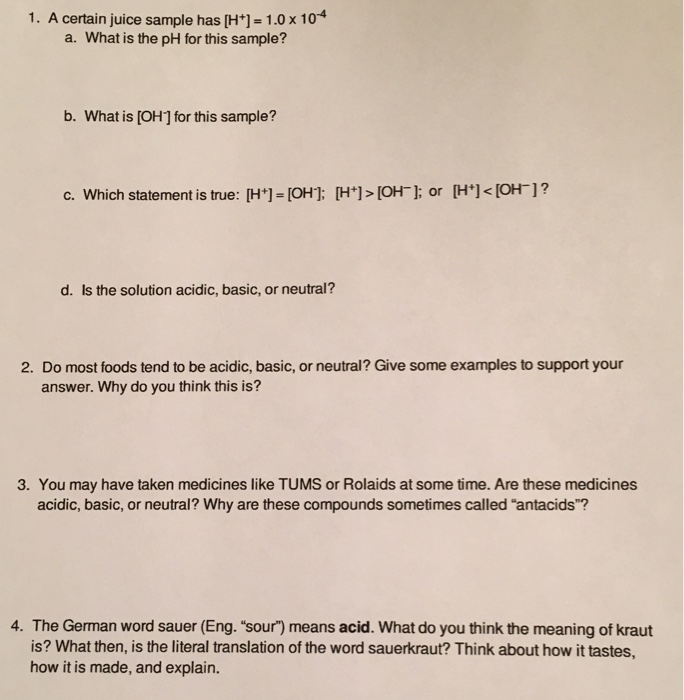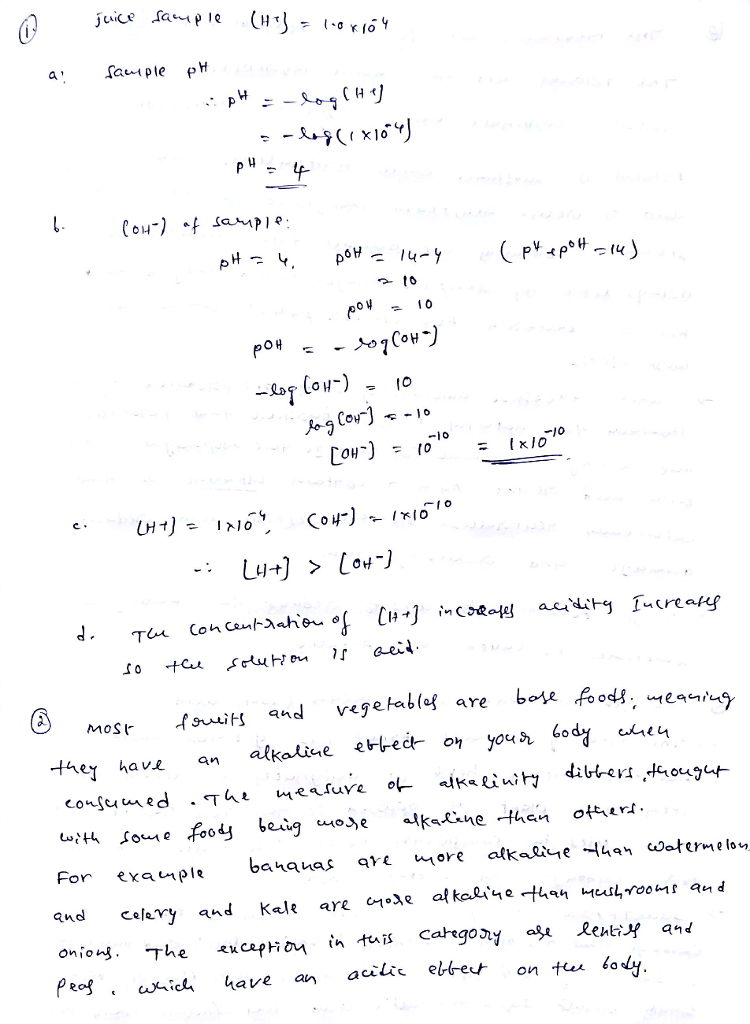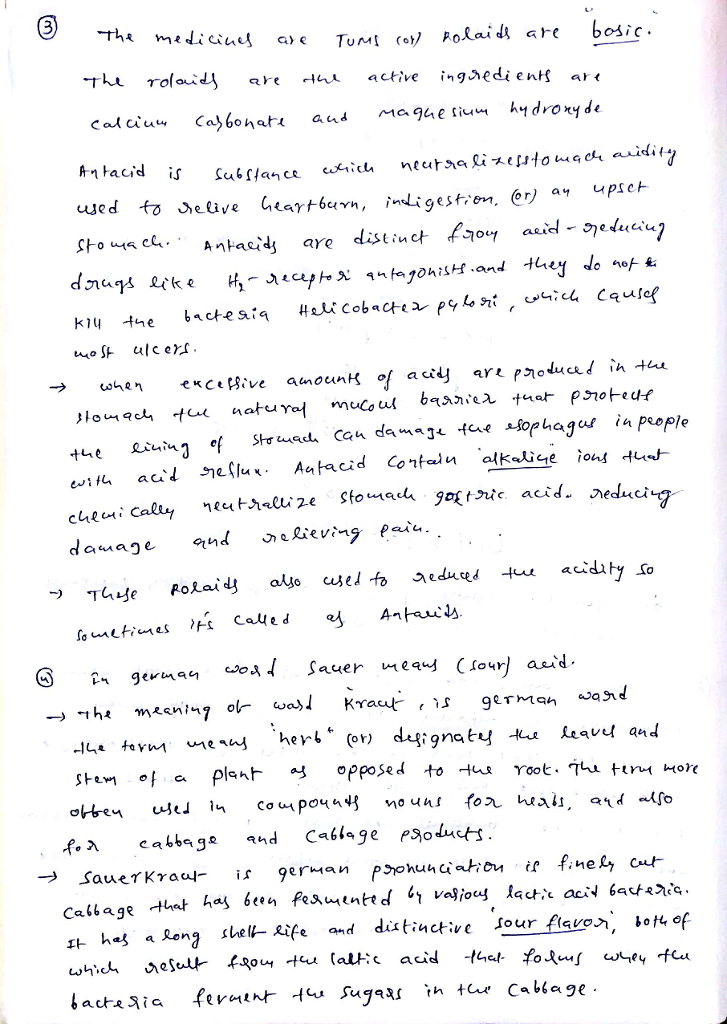# Question & Answer: A certain juice sample has [H^+] = 1.0 times 10^-4 a. What is the pH for this sample? b. What…..A certain juice sample has [H^+] = 1.0 times 10^-4 a. What is the pH for this sample? b. What is [OH] for this sample? c. Which statement is true: [H^+] = [OH^-] = [H^+] > [OH^-]: or [H^+]